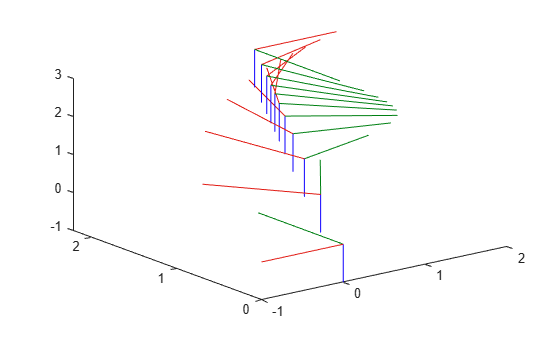Main Content

# Use Custom Time Scaling for a Transform Trajectory

This example shows how to specify custom time-scaling in the Transform Trajectory block to execute an interpolated trajectory. Two transformations are specified in the block to generate a trajectory between the two. The goal is to move between transforms using a nonlinear time scaling where the trajectory moves quickly at the start and slowly at the end.

### Open the Model

A custom time scaling trajectory is generated using the Polynomial Trajectory block, which gives the position, velocity, and acceleration defined by the custom time scaling at the instant in time, as given by the Clock block. The Clock block outputs simulation time and is used for querying the transformation trajectory at those specify time points. The input Waypoints define the waypoints of the nonlinear time scaling to use and includes a shorter time interval between points near the final time. The 3x1 time scaling, output from the Polynomial Trajectory block as q, qd, and qdd, is input to the Transform Trajectory block with the current clock time as the TSTime, which indicates this is the time scaling at that instance. The MATLAB® function block uses `plotTransforms` to plot a coordinate frame that moves along the generated transformation trajectory.

`open_system("custom_time_scaling_transform")`### Simulate the Model

Simulate the model. The plot shows how the transformation follows a nonlinear interpolated trajectory parameterized in time. The model runs with a fixed-step solver at an interval of 0.1 seconds, so each frame is 0.1 seconds apart. Notice that the transformations are sampled more closely near the final transformation.

`sim("custom_time_scaling_transform")`